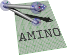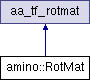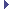amino  1.0-beta2 Lightweight Robot Utility Library
amino::RotMat Struct Reference

A rotation matrix object. More...

#include <tf.hpp>

Inheritance diagram for amino::RotMat:## Public Member Functions

RotMat ()
Construct an identity rotation matrix.

RotMat (const aa_tf_quat *p)
Construct a rotation matrix from a unit quaternion.

RotMat (const aa_tf_quat &p)
Construct a rotation matrix from a unit quaternion.

RotMat (const aa_tf_rotmat *p)
Construct a rotation matrix from another rotation matrix.

RotMat (const aa_tf_rotmat &p)
Construct a rotation matrix from another rotation matrix.

RotMat (const aa_tf_axang *p)
Construct a rotation matrix from an axis-angle.

RotMat (const aa_tf_axang &p)
Construct a rotation matrix from an axis-angle.

RotMat (const XAngle &p)
Construct a rotation matrix from a rotation about X.

RotMat (const YAngle &p)
Construct a rotation matrix from a rotation about Y.

RotMat (const ZAngle &p)
Construct a rotation matrix from a rotation about Z.

RotMat (double r11, double r12, double r13, double r21, double r22, double r23, double r31, double r32, double r33)
Construct a rotation matrix from individual components.

## Static Public Member Functions

static aa_tf_rotmat from_rotmat (double r11, double r12, double r13, double r21, double r22, double r23, double r31, double r32, double r33)
Create a rotation matrix from individual components.

static aa_tf_rotmat from_quat (const double x)
Create a rotation matrix from a unit quaternion.

static aa_tf_rotmat from_rotmat (const double x)
Create a rotation matrix object from a rotation matrix array.

static aa_tf_rotmat from_axang (const double x)
Create a rotation matrix from an axis-angle.

static aa_tf_rotmat from_rotvec (const double x)
Create a rotation matrix from a rotation vector.

static aa_tf_rotmat from_xangle (const double v)
Create a rotation matrix from a rotation about X.

static aa_tf_rotmat from_yangle (const double v)
Create a rotation matrix from a rotation about Y.

static aa_tf_rotmat from_zangle (const double v)
Create a rotation matrix from a rotation about Z.Public Attributes inherited from aa_tf_rotmat
union {
struct {
struct aa_tf_vec3   col0
column 0

struct aa_tf_vec3   col1
column 1

struct aa_tf_vec3   col2
column 2

}

double   data 
data array

};

## Detailed Description

A rotation matrix object.

Definition at line 313 of file tf.hpp.

The documentation for this struct was generated from the following file: